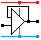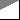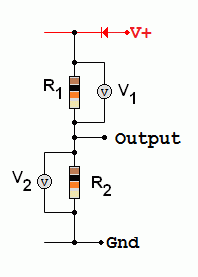Home

rOm
Quest
Glossary

Random
Page
Search
Site
Lush
Sim
Class
Subject
Images

Help
FAQ
Sign
Up
Log
In
 GCSE     Resistors     BS1852     Colour Codes     E24     LDR     Parallel     PIVR Calculations     Potentiometer     Puzzles     Series     Thermistor     Timing     Types     >Voltage Divider<

# Resistors Voltage Divider

Site for Eduqas/WJEC - Go to the AQA site.

GCSE    ResistorsQuestions 0 to 27   -->  View All

This circuit divides the voltage in the same ratio as the resistors so V1 / V2   =   R1 / R2   and   V2   =  Vs R2 / (R1 + R2)    where Vs is the supply voltage.R1 and R2 form a Voltage Divider. Usually one of these is variable and used to sense light, temperature, position, pressure etc.

The light sensing circuit turns on the lamp when it gets dark. A Schmitt trigger NOT gate should be used.

The voltage is divided in the ratio of the resistor values (assuming the current is the same in both resistors).

Two or more resistors in series can be used to set a required voltage at a point in a circuit. For example, this could correspond to

• a threshold voltage at which an alarm should sound
• the voltage output required from a power supply circuit
• the volume needed from a radio
• the speed at which a fan runs.

V2 = Vs R2 / (R1 + R2)    where Vs is the supply voltage.

V1 = Vs R1/ (R1 + R2)    where Vs is the supply voltage.

V1 / R1 = V2 / R2        or        V1 / V2 = R1 / R2        also        V1 + V2 = Vs

In this simulation, the resistors add up to 12kΩ and the voltage is 12 Volts.
This makes the maths stupidly simple.
Each "k" of resistance has one volt across it. Simples!
Click the switch to turn on the circuit.

Subject Name     Level     Topic Name     Question Heading     First Name Last Name Class ID     User ID

 CloseEscapeX Q: qNum of last_q     Q ID: Question ID         Score: num correct/num attempts         Date Done

Question Text

image url

Help Text
Debug

• You can attempt a question as many times as you like.
• If you are logged in, your first attempt, each day, is logged.
• To improve your scores, come back on future days, log in and re-do the questions that caused you problems.
• In the grade book, you can delete your answers for a topic before re-doing the questions. Avoid deleting unless you intend re-doing the questions very soon.